# DC/DC converter datasheets - Quiescent current demystified: Part two

At the end of part 1, I started talking about the no-load input supply current. But there is one more “quiescent” current that you need to look at before I continue.

Many DC/DC converters have an internal low dropout regulator (LDO) that supplies power to the converter’s internal circuits. In modern regulators, the input of the LDO is often available as an external pin on the converter. It is usually called “bias,” but check the data sheet to be sure that you have the right pin. With this input connected to the output of the regulator, the bias current acts as an extra load on the output of the converter. The load is down-converted just like any other load by the ratio of input voltage to output voltage. This is the preferred connection, since it reduces the current at the input and therefore improves efficiency.

Now let’s get back to the no-load input current. Sometimes you may not find this input current in the data sheet, or it is not specified under the conditions you’re interested in. In that case, you can use Equation 1 to estimate the no-load input current for a buck regulator: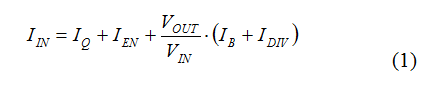This equation gives you a best-case estimate, since it does not take into account the losses in the converter. The first term, labeled IQ, is the non-switching quiescent current I talked about in the previous installment. The next term, IEN, is the current going into the regulator’s enable input. Many converters require a finite amount of bias current flowing into the enable input. If the enable pin is connected to the input supply to turn on the regulator, then you must account for that current; otherwise it is zero. The IDIV term is the current in the feedback divider and is easily calculated using Equation 2: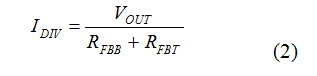The term IB represents the current flowing in the bias input that I just talked about. Let’s use the SIMPLE SWITCHER® LM43603 3.5V to 36V, 3A synchronous step-down voltage converter as an example. The data sheet has these typical values (Table 1).

If you are converting a 12V input to a 3.3V output, with a total feedback divider resistance of 1MΩ, Equations 1 and 2 give you the following value for the no-load input current: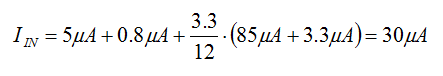Notice that, for the LM43603, the data sheet presents the no-load input current at one typical condition, as shown in Table 1.

My calculated value of 30µA is somewhat different, but it is close enough for a rough estimate.

The equations here are meant to show you how the no-load input current depends on the input voltage, output voltage and other “quiescent” currents. You can see that the input current will increase for larger output voltages and smaller input voltages. So the best thing to do is to use the equations to estimate the no-load input current and then measure the actual value under the real application conditions.

You can also calculate the input current of a DC/DC converter by using the efficiency curves, but not at no load. By definition the efficiency is zero at no load, so you must use the method I’ve outlined in this post to estimate the no-load supply current. At any other load, you can use the efficiency curves in the data sheet, along with Equation 3: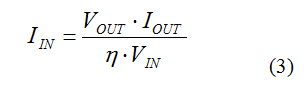where η is the efficiency under the conditions of interest.

Taking an example from the SIMPLE SWITCHER LM22670 3A step-down voltage regulator data sheet, the efficiency is about 91% for an input voltage of 5.5V and an output voltage of 3.3V at a load of 1.5A. That gives you an input current of about: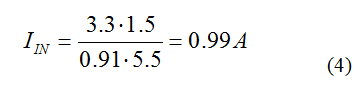Using and estimating the input currents found in data sheets is not difficult, as long as you make sure that the values you are looking at are applicable to your specific application conditions.